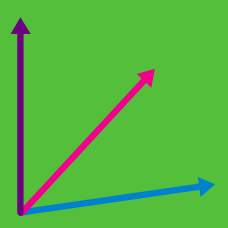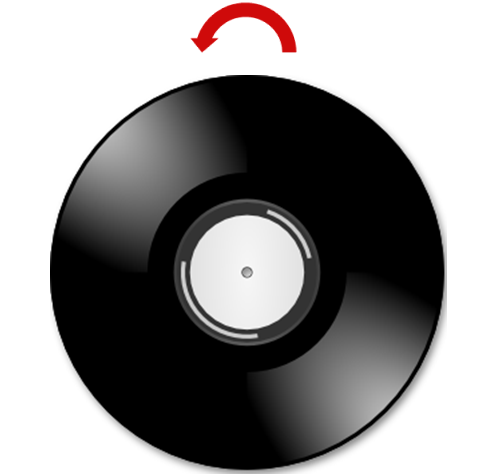Geometry

# Cross Product - Properties

Consider a vector $\vec{v}$ of magnitude $\sqrt{26}.$ If this vector is perpendicular to both $\vec{a}=(2,3,1)$ and $\vec{b}=(1,3,5),$ what is the square of its $x$-coordinate?

Given two vectors such that $\lvert\vec{a}\rvert=3,\lvert\vec{b}\rvert=4,$ and $\vec{a}\cdot\vec{b}=8,$ what is the magnitude of $\vec{b}\times\vec{a}?$Torque is vector that measures the tendency of a force to rotate an object about an axis. It is given by the formula $\vec{\tau}=\vec{r}\times\vec{F},$ where $\vec{r}$ is the displacement vector (pointing from the axis to the point at which the force is applied) and $\vec{F}$ is the force vector. If a disc rotates counter-clockwise on a record player, as shown in the figure above, what is the direction of the torque?

Find the volume of the parallelepiped spanned by the vectors $\vec{a}=(1,1,2), \vec{b}=(2,1,3),$ and $\vec{c}=(3,4,1).$

What is the volume of the tetrahedron whose vertices are $O=(0,0,0), A=(4,0,1), B=(-2,-4,3),$ and $C=(3,-1,-1)?$

×